# Comparative Balance Sheet _Class 12

A comparative balance sheet presents side-by-side information about an entity’s assets, liabilities, and shareholders’ equity as of multiple points in time. It shows increases and decreases in the various assets, liabilities and capital. A comparative balance sheet usually has two columns of amounts that appear to the right of the descriptions. The first column of amounts contains the amounts as of a recent moment or point of time i.e. current year and the column to the right containing corresponding amounts from a previous year.

Advantages of comparative balance sheet are:

1. The emphasis of comparative balance sheet is on change.
2. It acts as a connection between Statement of Profit and Loss and Balance Sheet.
3. A comparative balance sheet is helpful in comparison of a single year’s balance sheet.

#### Comparative Balance Sheet : Example 1

Following is the information provided by A LTD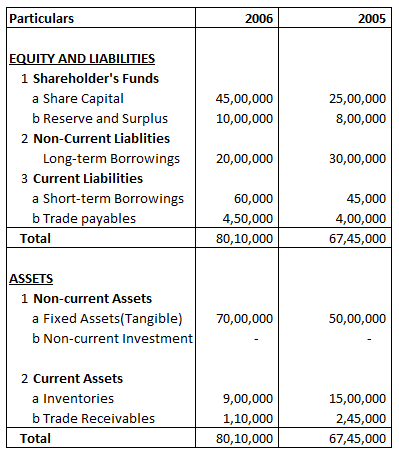Absolute Change and Percentage Change in share capital is

Explanation : –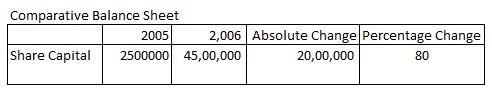Absolute Change = Current year’s figure – Previous year’s figure
= 4500000 – 2500000
= 2000000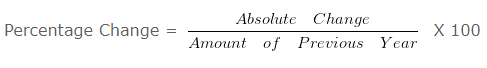= 2000000/2500000 X 100

= 80 %

#### Comparative Balance Sheet : Example 2

Following is the Balance sheet of AA Ltd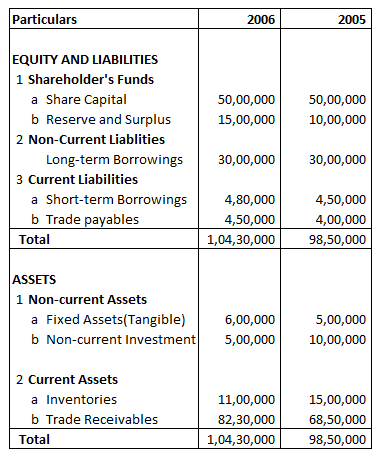Absolute Change and Percentage Change in Reserve and Surplus is:

Explanation : –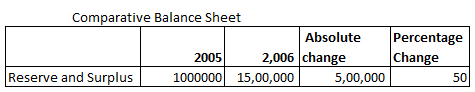Absolute change = Current year’s figure – Previous year’s figure
= 1500000 – 1000000
= 500000= 500000/1000000 X 100

= 50

#### Comparative Balance Sheet : Example 3

Following is the Balance sheet of B Ltd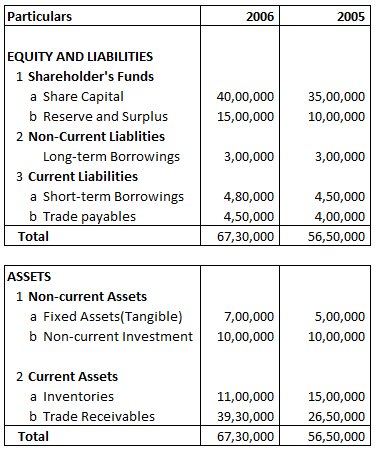Absolute Change and Percentage Change in Long-term Borrowings is:

Explanation : –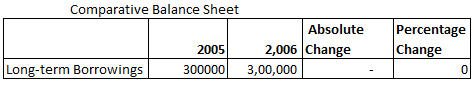Absolute Change = Current year’s figure – Previous year’s figure
= 300000 – 300000
= 0= 0/300000 X 100

= 0

#### Comparative Balance Sheet : Example 4

Following is the Balance sheet of A Ltd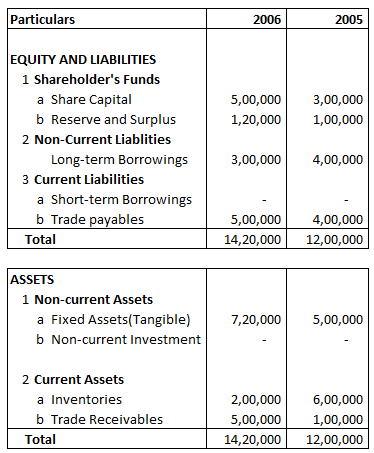Absolute Change and Percentage Change in Trade payable is:

Explanation : –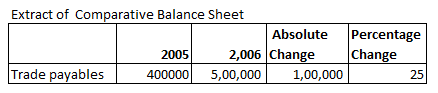Absolute Change = Current year’s figure – Previous year’s figure
= 500000 – 400000
= 100000= 100000/400000 X 100

= 25

#### Comparative Balance Sheet : Example 5

Following is the Balance sheet of A Ltd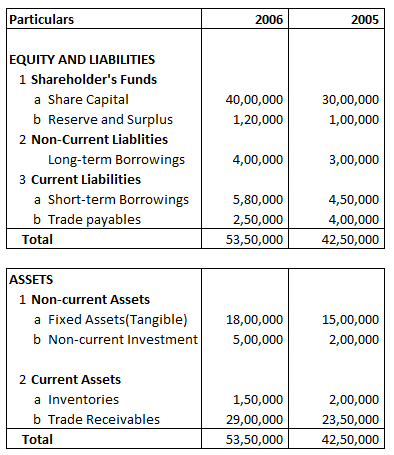Absolute Change and Percentage Change in Fixed Assets(Tangible) is:

Explanation : –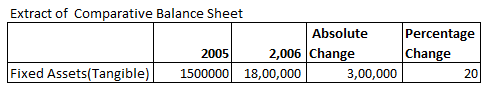Absolute Change = Current year’s figure – Previous year’s figure
= 1800000 – 1500000
= 300000= 300000/1500000 X 100

= 20

#### Example 6

Following is the Balance Sheet of AX Ltd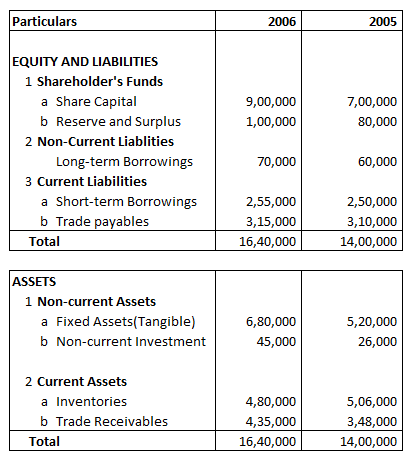Share Capital Percentage of Balance Sheet total for 2005 will be:

Explanation : –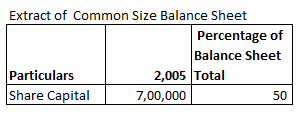Share Capital Percentage of Balance Sheet Total 2005 =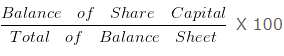= 700000/1400000 X 100

= 50

#### Comparative Balance Sheet : Example 7

Following is the Balance Sheet of A LtdReserve and Surplus Percentage of Balance Sheet total for 2005 will be:

Explanation : –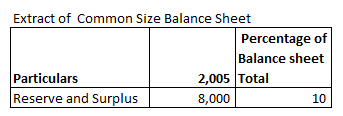Reserve and Surplus Percentage of Balance Sheet Total 2005 =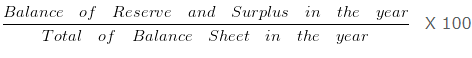= 8000/80000 X 100

= 10

#### Comparative Balance Sheet : Example 8

Following is the balance sheet of A Ltd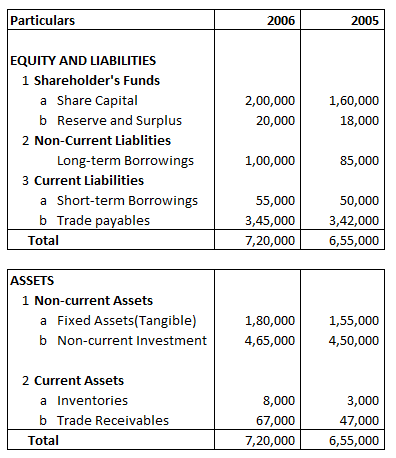Fixed Assets(Tangible) Percentage of Balance Sheet Total for 2006 will be:

Explanation : –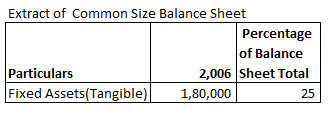Fixed Assets(Tangible) Percentage of Balance Sheet Total 2006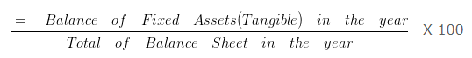= 180000/720000 X 100

= 25

#### Example 9

Following is the Balance sheet of AB Ltd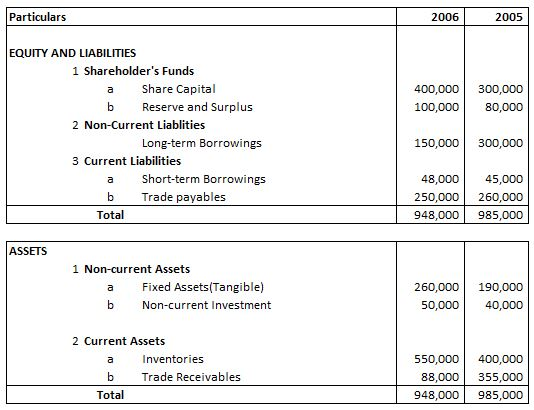Absolute Change and Percentage Change in Inventories is:

Explanation : –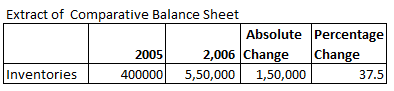Absolute Change = Current year’s figure – Previous year’s figure
= 550000 – 400000
= 150000= 150000/400000 X 100

= 37.5